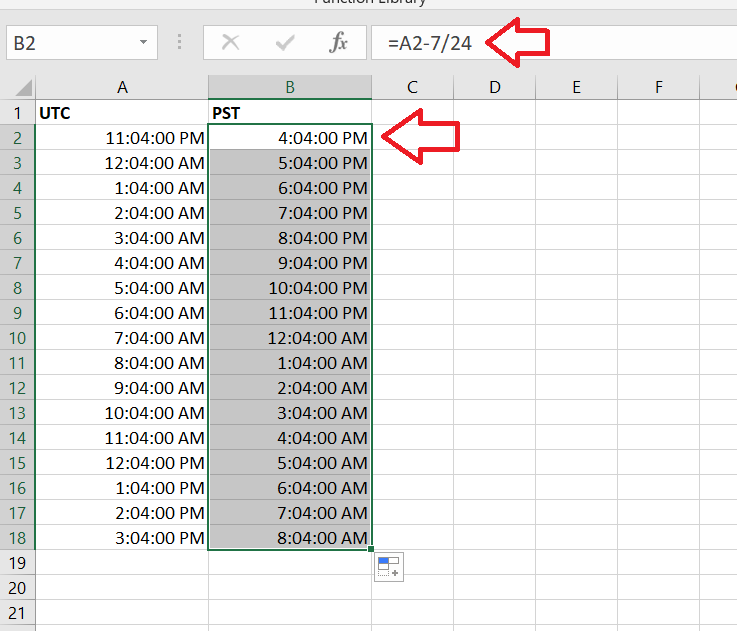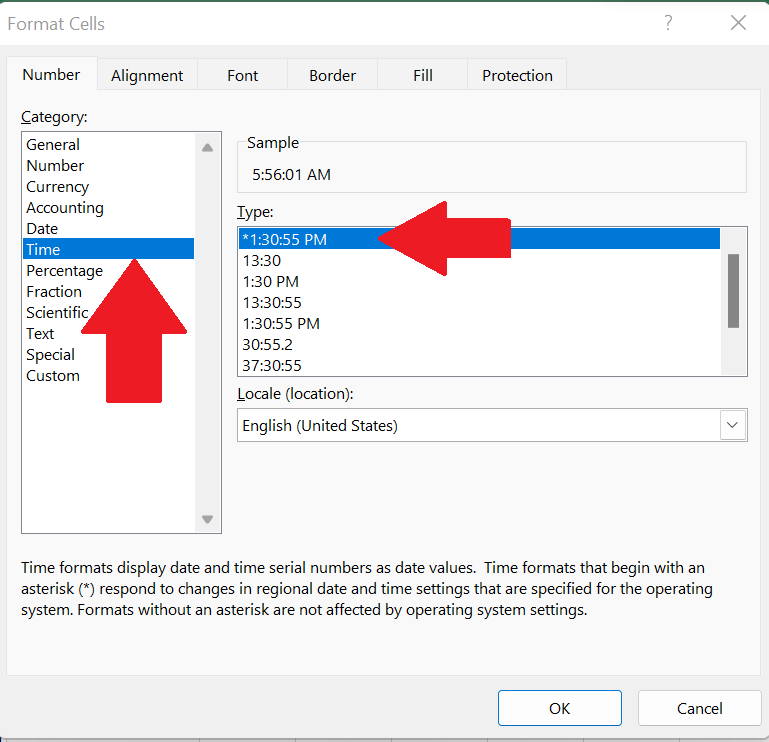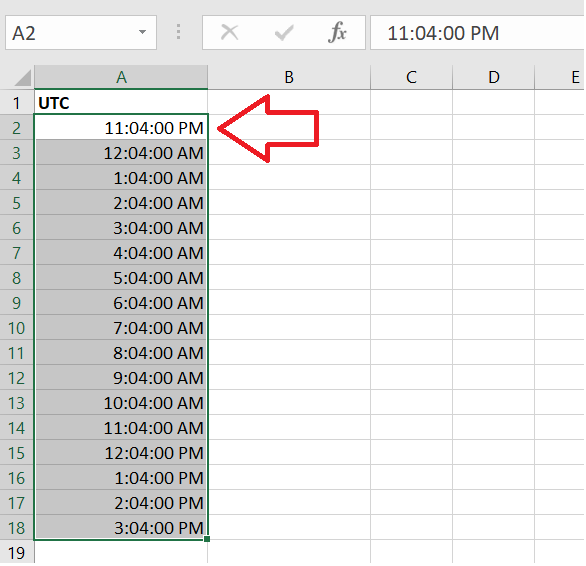# UTC to PST Converter in Excel

So let’s see how to convert the time zone of UTC(Universal Time Coordinated) to PST(Pacific Standard Time). Before moving ahead we should know the time difference between the two time zones in whole numbers, e.g PST is 8 hours behind UTC. Which means that the offset between and PST  will be 8 or PST is equal to UTC  – 8:00 hours. We will consider the difference – 7, because the one hour difference is due to PDT(Pacific Daylight Time). Now follow along the steps to create a simple time zone convertor in Excel.Microsoft Excel is a software that can also help us in mathematical calculations along with data analysis. There are amazing features of Microsoft Excel which helps a lot in day to day routine. For example, it can help us in time zone conversion too with a very simple method.

### Step-1 Select the Cell Format– Open Excel and select the blank sheet.

– Choose a suitable cell where you wish to type the UTC time. In this case we have chosen A2.

– Press the shortcut key Ctrl + 1 to set the cell format to TIME from General.

– A dialogue box will appear, select the cell type as TIME, then select the type given at the right side.

– Click OK as shown in picture below;

### Step 2 – Type the UTC time– Type in any time value that you wish to convert from UTC to PST in A2.

– Drag it down a few rows to create some data samples.

– Excel will automatically increment in hours.

### Step 3 – Implement the formula– Choose a suitable cell where you wish to implement the formula. In this case we have chosen B2 to implement this formula.

– As UTC is 7 hours behind PST. We will subtract 7 hours from UTC time.

– We will divide with 24 for A.M/P.M conversion.

– Therefore, = A2 – 7 / 24 will be the formula to be used in the cell B2.

– The desired result will be displayed in this cell as soon as you press the enter key.

– Drag down with the handle till the last range of time in column A.

– All the time values of UTC in column A are converted to PST and displayed in column B, just like the picture shown below.

In the formula bar, you can see the formula. After dragging it down , all time ranges have been automatically calculated and displayed.